# GSEB Solutions Class 11 Maths Chapter 6 Linear Inequalities Ex 6.1

Gujarat Board GSEB Textbook Solutions Class 11 Maths Chapter 6 Linear Inequalities Ex 6.1 Textbook Questions and Answers.

## Gujarat Board Textbook Solutions Class 11 Maths Chapter 6 Linear Inequalities Ex 6.1

1. Solve 24x < 100, when
(i) x is a natural number.
(ii) x is an integer.
Solution:
Dividing by 24, we get
x < $$\frac{100}{24}$$ = $$\frac{25}{6}$$ This inequality is true, when (i) x = 1, 2, 3, 4 [x being a natural number] (ii) Solution of inequality is …, – 4, – 3, – 2, – 1, 0, 1, 2, 3, 4 (x being an integer).2. Solve – 12x > 30, when
(i) x is a natural number,
(ii) x is an integer.
Solution:
– 12x > 30.
Dividing by -12. we get
x < – $$\frac{30}{12}$$ = $$\frac{- 5}{2}$$.
(i) This inequality is not true for any natural number.
(ii) Integers that satisfy this inequality are ,…, – 5, – 4, – 3.3. Solve 5x – 3 < 7, when
(i) x is a natural number
(ii) visa real number.
Solution:
5x – 3 < 7
Transposing 3 to R.H.S., we get
5x < 7 + 3 or 5x < 10
Dividing by 2, we get,
x < 2.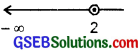(i) When x is a natural number.
x = 1 satisfies this inequality.
(ii) When x is a real number, the solutions are (- ∞, 2).

4. Solve 3x + 8 > 2, when
(i) x is an integer
(ii) x is a real number.
Solution:
Inequality is 3x + 8 > 2.
Transposing 8 to R.H.S., we get
3x > 2 – 8 = – 6.
Dividing by 3, we get
x > – 2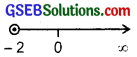(i) When x is an integer, the solutions are – 1, 0, 1, 2, 3, …
(ii) When x is real number, the solutions are (- 2, ∞).Solve the following inequalities in questions 5 to 16 for real x:
5. 4x + 3 < 6x + 7 6. 3x – 7 > 5x – 1
7. 3(x – 1) ≤ 2(x – 3)
8. 3(2 – x) ≥ 2(1 – x)
9. x + $$\frac{x}{2}$$ + $$\frac{x}{3}$$ < 11
10. $$\frac{x}{3}$$ > $$\frac{x}{2}$$ + 1
11. $$\frac{3(x – 2)}{5}$$ ≤ $$\frac{5(2 – x)}{3}$$
12. $$\frac{1}{2}$$($$\frac{3x}{5}$$ + 4) ≥ $$\frac{1}{3}$$(x – 6)
13. 2(2x + 3) – 10 < 6(x – 2)
14. 37 – (3x + 5) ≥ 9x – 8(x – 3)
15. $$\frac{x}{4}$$ < $$\frac{(5x – 2)}{3}$$ – $$\frac{(7x – 3)}{5}$$
16. $$\frac{(2x – 1)}{3}$$ ≥ $$\frac{(3x – 2)}{4}$$ – $$\frac{(2 – x)}{5}$$
Solutions for questions 5 to 16:
5. The inequality is 4x + 3 < 6x + 7.
Transposing 6x to L.H.S. and 3 to R.H.S., we get
4x – 6x < 7 – 3.
or – 2x < 4.
Dividing by – 2, we get x > – 2
∴ The solution is (- 2, ∞).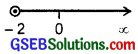6. The inequality is 3x – 7 > 5x – 1.
Transposing 5x to L.H.S. and – 7 to R.H.S., we get
3x – 5x > -1 + 7.
or – 2x > 6.
Dividing by – 2, we get
x < – 3
∴ The solution is (- ∞, – 3).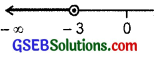7. The inequality is
3(x – 1) ≤ 2(x – 3).
On simplifying, we get
3x – 3 ≤ 2x – 6
Transposing 2x to L.H.S. and – 3 to R.H.S., we get
3x – 2x ≤ – 6 + 3.
or x ≤ – 3.
∴ The solution is (- ∞, – 3],8. The inequality is
3(2 – x) ≥ 2(1 – x).
Simplifying, we get
6 – 3x ≥ 2 – 2x.
Transposing – 2x to L.H.S. and 6 to R.H.S., we get
– 3x + 2x ≥ 2 – 6.
or – x ≥ – 4.
Multiplying by – 1, we get
x ≤ 4.
∴ The solution is (- ∞, 4],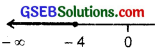9. The inequality is
x + $$\frac{x}{2}$$ + $$\frac{x}{3}$$ < 11.
Simplifying, we get$$\frac{6x+3x+2x}{6}$$ < 11.
or $$\frac{11x}{6}$$ < 11.
Dividing both sides by $$\frac{11}{6}$$, we get
x < 6. ∴ The solution is (- ∞, 6).10. The inequality is $$\frac{x}{3}$$ > $$\frac{x}{2}$$ + 1.
Transposing $$\frac{x}{2}$$ to L.H.S., we get
$$\frac{x}{3}$$ – $$\frac{x}{2}$$ > 1.
⇒ $$\frac{2x-3x}{6}$$ > 1.
or – $$\frac{x}{6}$$ > 1.
Multiply by – 6, we get
x < – 6.
∴ The solution is (- ∞, – 6).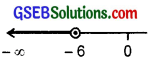11. The inequality is
$$\frac{3(x – 2)}{5}$$ ≤ $$\frac{5(2 – x)}{3}$$.
Multiply both sides by L.C.M. of 5 and 3, i.e., by 15.
3 × 3(x – 2) ≤ 5 × 5(2 – x).
or 9(x – 2) ≤ 25(2 – x).
Simplifying, we get
9x – 18 ≤ 50 – 25x.
Transposing – 25x to L.H.S. and – 18 to R.H.S., we get
∴ 9x + 25x ≤ 50 + 18.
or 34x ≤ 68
Dividing by 34, we get
x ≤ 2.
∴ Solution is (- ∞, 2].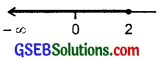12. The inequality is
$$\frac{1}{2}$$($$\frac{3x}{5}$$ + 4) ≥ $$\frac{1}{3}$$(x – 6).
or $$\frac{1}{2}$$($$\frac{3x + 20}{5}$$ ≥ $$\frac{1}{3}$$(x – 6).
Multiplying both sides by 30, we get3(3x + 20) ≥ 10(x – 6)
or 9x + 60 ≥ 10x – 60.
Transposing 10x to L.H.S. and 60 to R.H.S., we get
9x – 10x ≥ – 60 – 60
or – x ≥ – 120.
Multiplying by – 1, we get
x ≤ 120.
∴ The solution is (- ∞, 120].

13. The inequality is
2(2x + 3) – 10 < 6(x – 2).
Simplifying, we get
4x + 6 – 10 < 6x – 12
or 4x – 4 < 6x – 12.
Transposing 6x to L.H.S. and – 4 to R.H.S., we get
4x – 6x < – 12 + 4
or – 2x < – 8. Dividing by – 2, we get x > 4.
∴ The solution is (4, ∞).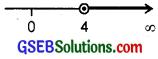14. The inequality is
37 – 3x – 5 ≥ 9x – 8 + 24
or 32 – 3x ≥ x + 24.
Simplifying, we get
37 – 3x – 5 ≥ 9x – 8x + 24
or 32 – 3x ≥ x + 24.
Transposing x to L.H.S. and 32 to R.H.S., we get
– 3x – x ≥ 24 – 32.
or – 4x ≥ – 8.
Dividing by – 4, we get
x ≤ 2.
∴ The solution is (- ∞, 2].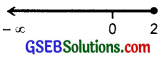15. The inequality is
$$\frac{x}{4}$$ < $$\frac{5x – 2}{3}$$ – $$\frac{7x – 3}{5}$$.
Multiplying each term by the L.C.M. of 4, 3 and 5, i.e., 60, we get
15x < 20(5x – 2) – 12(7x – 3)
Simplifying, we get
15x < 100x – 40 – 84x + 36
or 15x < 100x – 84x – 40 + 36
15x < 16x – 4,
Transposing 16x to L.H.S., we get
15x – 16x < – 4
or – x < – 4. Multiplying by – 1, we get x > 4.
∴ The solution is (4, ∞).16. The inequality is
$$\frac{2x – 1}{3}$$ ≥ $$\frac{3x – 2}{4}$$ – $$\frac{2 – x}{5}$$.
Multiplying each term by L.C.M. of 3, 4 and 5, i.e., 60, we get
$$\frac{2x – 1}{3}$$ × 60 ≥ $$\frac{3x – 2}{4}$$ × 60 – $$\frac{2 – x}{5}$$ × 60
or 20(2x – 1) ≥ (3x – 2) × 15 – (2 – x) × 12.
or 40x – 20 ≥ 45x – 30 – 24 + 12x.
or 40x – 20 ≥ 57x – 54.
Transposing 57x to L.H.S. and – 20 to R.H.S., we get
40x – 57x ≥ – 54 + 20.
or – 17x ≥ – 34
Dividing by – 17, we get
x ≤ 2.
∴ The solution is (- ∞, 2).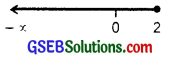17. The inequality is
3x – 2 < 2x + 1.
Transposing 2x to L.H.S. and – 2 to R.H.S., we get
3x – 2x < 1 + 2
or x < 3.
The solution is (- ∞, 3). Also, see the number line.18. The inequality is
5x – 3 ≥ 3x – 5.
Transposing 3x to L.H.S. and – 3 to R.H.S., we get
∴ 5x – 3x ≥ – 5 + 3
or 2x ≥ – 2
or x ≥ – 1.
∴ The solution is (- 1, ∞). Also, see number line.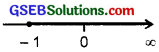19. The inequality is
3(1 – x) < 2(x + 4).
Simplifying, we get
3 – 3x < 2x + 8
Transposing 2x to L.H.S. and 3 to R.H.S., we get
– 3x – 2x < 8 – 3
or – 5x < 5. Dividing both sides by – 5, we get x > – 1.
∴ The solution is (- 1, ∞). Also, see number line.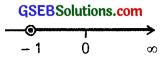20. The inequality is
$$\frac{x}{2}$$ < $$\frac{5x – 2}{3}$$ – $$\frac{7x – 3}{5}$$.
Multiply each term by L.C.M. of 2, 3 and 5, i.e., by 30, we get
$$\frac{x}{2}$$ × 30 < $$\frac{5x – 2}{3}$$ × 30 – $$\frac{7x – 3}{5}$$ × 30.
or 15x < 10(5x – 2) – 6(7x – 3)
or 15x < 50x – 20 – 42x + 18
or 15x < 8x – 2.
Transposing 8x to L.H.S., we get
15x – 8x < – 2
or 7x < – 2.
Dividing by 7, we get
x < – $$\frac{2}{7}$$.
∴ The solution is (- ∞, – $$\frac{2}{7}$$).
Also, see the number line.21. Ravi obtained 70 and 75 marks in first two unit tests. Find the number if minimum marks he should get in the third test to have an average of at least 60 marks?
Solution:
Let Ravi gets x marks in third unit test.
∴ Average marks obtained by Ravi
= $$\frac{70 + 75 + x}{3}$$.
He is to obtain at least 60 marks.
∴ $$\frac{70 + 75 + x}{3}$$ ≥ 60.
or $$\frac{145 + x}{3}$$ ≥ 60.
Multiplying by 3, we get
145 + x ≥ 60 × 3 = 180.
Transposing 145 to R.H.S., we get
x ≥ 180 – 145
= 35.
∴ Ravi should get at least 35 marks in the third unit test.

22. To receive ‘grade A’ in a course, one must obtain an average of 90 marks or more in five examinations (each of 100 marks). If Sunita’s marks in first four examinations are 87, 92, 94 and 95, find minimum marks that Sunita must obtain in fifth examination to get grade ‘A’ in the course?
Solution:
Let Sunita obtains x marks in the fifth examination.
∴ Average of marks of 5 examinations
= $$\frac{87+92+94+95+x}{5}$$
= $$\frac{368+x}{5}$$.
This average must be at least equal to 90.
So, $$\frac{368+x}{5}$$ ≥ 90.
or 368 + x ≥ 5 × 90 = 450
or x ≥ 450 – 368 = 82.
i.e., she should obtain at least 82 marks in the fifth examination.23. Find all pairs of consecutive odd positive integers both of which are smaller than 10, such that their sum is more than 11?
Solution:
Let x be the smaller of the two odd positive integers so that the other is x + 2. Then, we should have:
x < 10. ∴ x + (x + 2) > 11
or 2x + 2 > 11
or 2x > 11 – 2
or 2x > 9
or x > $$\frac{9}{2}$$.
Hence, if one number is 5 (odd number), then the other is seven, since 7 < 10, if the smaller number is seven, then the other is nine. Hence, possible pairs are (5, 7) and (7, 9).

24. Find all pairs of consecutive even positive integers, both of which are larger than 5, such that their sum is less than 23? Solution: Let x be the smaller of the two positive even integers. Then, the other one is x + 2. Then we should have: x > 5
and x + x + 2 < 23.
or 2x + 2 < 23.
Since if the sum is less than 23, then it is also less than 24.
∴ or 2x + 2 < 24
or 2x < 22.
or x < 11
Thus, the value of x may be 6, 8, 10 (even integer).
Hence, the pairs may be (6, 8), (8, 10), (10, 12).25. The longest side of a triangle is 3 times the shortest side and the third side is 2 cm shorter than the longest side. If the perimeter of the triangle is at least 61 cm, find the minimum length of the shortest side?
Solution:
Let shortest side measure x cm.
∴ The longest side will be 3x cm.
∴ Third side will be (3x – 2) cm.
According to the problem,
x + 3x + 3x – 2 ≥ 61.
or 7x – 2 ≥ 61
or 7x ≥ 63.
or x ≥ 9 cm.
Hence, the minimum length of the shortest side is 9 cm.
Note the other sides measure 27 cm and 25 cm.

26. A man wants to cut three lengths from a single piece of board of length 91 cm. The second length is to be 3 cm longer than the shortest and the third length is to be twice as long as the shortest. What are the possible lengths for the shortest board, if the third piece is to be at least 5 cm longer than the second?
Solution:
Let x be the length of tlie shortest board. Then, x + 3 is the second length and 2x is the third length. Thus,
x + (x + 3) + 2x ≤ 91.
or 4x + 3 ≤ 91
or 4x ≤ 88
or x ≤ 22.
According to the problem
2x ≥ (x + 3) + 5
or x ≥ 8.
So, shortest board should be at least 8 cm long but not more than 22 cm long.